## Business Analytics - Assignment 1

Compute the required amount of account managers and involved traveling distance for EMTE. EMTE is a supermarket chain in the Netherlands with about 130 stores. EMTE likes to know how many account managers they need to visit all the stores in one day, and how many kilometers they need to travel in total together. Their main objective is to minimize the total traveling distance rather than the amount of account managers. Every account manager starts and ends at the headquarters. Due to the maximum working time of the account manager, every account manager can drive a maximum of 400 km per day. In other words: the task is to solve a VRP for this problem. The goal is to minimize the total distance. You can use the haversine formula in Matlab or Python to compute the distance between two locations.### 1. Functions¶

These functions are used for exercises 1, 2, 3 and 4. Exercise 5 has its own set of functions due to having different constraints.

In :
import pulp
import collections
import time
import random
import folium
import pants
import math

import pandas              as pd
import numpy               as np
import matplotlib.pyplot   as plt

from copy                          import deepcopy
from haversine                     import haversine
from __future__                    import print_function
from simanneal                     import Annealer
from sklearn.metrics.pairwise      import pairwise_distances

def get_route_ilp(VRP):
""" Extract the routes from pulp

The function aims to extract the routes from
pulp as those are saved a bit messy.

Parameters:
-----------
VRP (pulp): The full VRP solution

Returns:
-----------
dict(int, list): dictionary of multiple routes

"""
temp_routes = {}

for v in VRP.variables():
if (v.varValue == 1) and ('Edge_' in str(v)):
route_number = int(str(v.name).split('_')[-1].split(')'))
from_city = int(str(v.name).split('(').split(','))
to_city = int(str(v.name).split('(').split('_').split(','))
if int(from_city) == 0:
temp_routes[route_number] = {}
temp_routes[route_number][from_city] = to_city
else:
temp_routes[route_number][from_city] = to_city

ilp_routes = {}

for route in temp_routes:
temp_route = 
for i in range(len(temp_routes[route])):
temp_route.append(temp_routes[route][temp_route[-1]])
ilp_routes[route] = [0, temp_route]

return ilp_routes

def get_distance_matrix(cities):
""" Create a numpy distance matrix for pulp

A numpy distance matrix is created of all the
cities in order for pulp to work with it.

Parameters:
-----------
cities (dataframe): Dataframe of all cities

Returns:
-----------
dist_matrix (numpy): Numpy distance matrix
"""
distance_matrix = np.zeros((cities.shape, cities.shape))

coordinates = cities[["Lat", "Long"]].values

for i in range(distance_matrix.shape):
for j in range(distance_matrix.shape):
distance_matrix[i][j] = haversine\
((coordinates[i],coordinates[i]),
(coordinates[j],coordinates[j]))

return distance_matrix

def get_distance_route(route):
"""
Get total distance for a single route
with HQ being both the start and finish

Parameters:
-----------
route (list) : List of route with cities as int.

Returns:
--------
total_distance (float): Distance in km.

Example:
--------
>>>> get_distance_route([0, 120, 3, 2, 0])
414.746557867148

"""

total_distance = 0

for i in range(len(route)-1):
city_1 = route[i]
city_2 = route[i+1]
total_distance += distances_dict[city_1][city_2]

def get_distance_routes(routes):
"""
Return the total distance for a set of routes
with HQ being both the start and finish

Parameters:
-----------
route (dict of list): Dict of lists of multiple routes
with cities as int.

Returns:
--------
total_distance (float): Total distance in km.

Example:
--------
>>>> get_distance_route(set_of_routes)
914.746557867148
"""

total_distance = 0

for i in routes:
route = routes[i]

for j in range(len(route)-1):
city_1 = route[j]
city_2 = route[j+1]
total_distance += distances_dict[city_1][city_2]

def nearest_neighbor(df):
""" Returns a list of routes based on an implementation
of the nearest neighbor heuristic. The routes have
the following constraints:
* Every route cannot be longer than 400km
* No subroutes are allowed
* All cities in a route are not visited in another route

Parameters:
-----------
df (dataframe): Distance matrix of distances between each city

Returns:
--------
routes (dictionary): Dictionary of lists which contain cities
in the correct order
"""
routes = {}

while len(df) > 1:
current_city = 0
total_distance = 0
route = [0, 0]

while True:
# Find closest city
new_city_distance = df.drop([current_city, 0], axis=1).ix[current_city].min()
new_city = int(df.drop([current_city, 0], axis=1).ix[current_city].idxmin())
distance_to_hq = df.ix[new_city]

# Check if "future" distance matches with current distance
if (total_distance + new_city_distance + distance_to_hq) < 400:

# Remove city from places to visit
if current_city != 0:
df = df.drop(current_city)
df = df.drop(current_city, axis = 1)

# Update Route and set the starting city to the closest one
current_city = new_city
route.insert(-1, int(new_city))
total_distance += new_city_distance

# If there is only 1 city left we take that as a single route
if len(df) == 2:
total_distance += distance_to_hq
routes[len(routes) + 1] = [total_distance, route]
df = df.drop(current_city)
break

# Signifies the end of a route
else:
total_distance += distance_to_hq
routes[len(routes) + 1] = [total_distance, route]
df = df.drop(current_city)
df = df.drop(current_city, axis = 1)
break

return routes

def plot_routes(routes, method = '', figure_size = (10, 8)):
""" Show a plot of all routes that are created with different colors

Parameters:
-----------
routes (dict of lists): Distance matrix of distances between each city
method (string): Assigns method to title
figure_size (tuple): Change figure size

"""

# Create figure with distance in title
sum_distance = sum([get_distance_route(routes[route]) for route in routes])
plt.figure(figsize=figure_size)
plt.title("Distance: {}. Method: {}".format(round(sum_distance, 4), method))

# Create subplot for each route
for route in routes:
xseries = [df.Long.iloc[index] for index in routes[route]]
yseries = [df.Lat.iloc[index] for index in routes[route]]
plt.scatter(xseries,yseries)
plt.plot(xseries,yseries,linestyle='-', marker='o')

# For easier visability
plt.xlim(3.3,7.1)
plt.ylim(51.0,53.15)
plt.show()

def fancy_plot(routes):
""" Show a fancy plot of all routes that are created with different colors
using folium to show the map of the Netherlands

Parameters:
-----------
routes (dict of lists): Distance matrix of distances between each city
"""
# Create list of (lat, long)
points = []
for i in range(len(df)):
points.append(tuple([df.Lat.values[i], df.Long.values[i]]))

# Create map with certain size
ave_lon = sum(p for p in points)/len(points)
ave_lat = 52.15
my_map = folium.Map(location=[ave_lat, ave_lon], zoom_start=7,
height = '80%', width = '80%')
points_routes = {j: [(df.Lat[i], df.Long[i]) for i in routes[j]]
for j in routes}

# Plot points onto map
colors = ['blue', 'green', 'red', 'black', 'purple', 'orange', 'brown']
for route in points_routes:
folium.PolyLine(points_routes[route], color = colors[route-1],

for point in points_routes[route]:
fill_opacity = 1, color = colors[route-1],
return my_map

def city_swap(route):
""" Single iteration of a consecutive city swap

City swap is implemented as a within-route consecutive
city swap. In other words, it tries to swap consecutive
cities in a single route and returns a route as soon
as it is better then the starting route.

Parameters:
-----------
route (list): Route with cities as integers in a list

Returns:
-----------
list(float, list): list(distance of top route, the top route)

"""
top_route = deepcopy(route)
top_route_distance = get_distance_route(route)

if len(route) > 3:
for i in range(1, len(route)-2):
temp_route = deepcopy(top_route)
temp_route[i], temp_route[i+1] = temp_route[i+1], temp_route[i]
if get_distance_route(temp_route) < top_route_distance:
top_route = temp_route

return [top_route_distance, top_route]

def city_swap_extended(routes):
""" A between route version of city swap

An extended version of the consecutive city swap.
This heuristic tries to swap each cities in a route
with the closest city in another route. It returns
as soon as it finds a solution that is better than
the initial solution.

Parameter:
-----------
routes (dict(int, list)): dictionary of multiple routes

Returns:
-----------
dict(int, list): dictionary of multiple routes

"""
# Initialize top route and distance
top_routes = deepcopy(routes)
top_distance = get_distance_routes(top_routes)

for route_1 in top_routes:
for route_2 in top_routes:
for ix_1, city in enumerate(top_routes[route_1]):
if (city != 0) & (route_1 != route_2) & (len(top_routes[route_2]) > 2):

# Find the closest city in another route
temp = deepcopy(top_routes)
city_2 = {key: distances_dict[city][key] for key in temp[route_2]}
city_2.pop(0, None)
city_2.pop(city, None)
city_2 = min(city_2, key=city_2.get)
ix_2 = temp[route_2].index(city_2)

# Swap the cities between routes
temp[route_1][ix_1], temp[route_2][ix_2] = temp[route_2][ix_2],\
temp[route_1][ix_1]
temp_distance = [get_distance_route(temp[i]) for i in temp]

# Check if it fits the constraints and better then initial solution
if (all(dist < 400 for dist in temp_distance)) and (sum(temp_distance) <\
top_distance):
top_routes = deepcopy(temp)
top_distance = temp_distance

def two_opt_step(route):
""" Single iteration of a two-opt exchange

This version of two-opt exchange is implemented as an within
route two-opt exchange. Thus, within a route, a piece of the
route is reversed in order to possibly optimize the route.
It returns as soon as it finds a better solution than the
initial route.

Parameters:
-----------
route (list): Route with cities as integers in a list

Returns:
-----------
list(float, list): list(distance of top route, the top route)

"""
top_route = route
top_route_distance = get_distance_route(route)

if len(route) > 2:
for i in range(1, len(route)-2):
for j in range(i+1, len(route)):
temp_route = route[:i] + route[i:j][::-1] + route[j:]
temp_route_distance = get_distance_route(temp_route)

if temp_route_distance < top_route_distance:
top_route = temp_route
top_route_distance = temp_route_distance
return [top_route_distance, top_route]

return [top_route_distance, top_route]

def two_opt_step_ext(routes):
""" Single iteration of a two-opt exchange

A single iteration of a two-opt exchange between
different routes. The heuristic takes two routes and
splits both of them in a random point to create the following
routes: 1A, 1B, 2A and 2B. Then, the routes are combined as
follows: (1A2A and 1B2B) or (1A2B, 1B2A). The algorithm checks
which one is better and returns as soon as the new route is
better than the initial route.

Parameter:
-----------
routes (dict(int, list)): dictionary of multiple routes

Returns:
-----------
dict(int, list): dictionary of multiple routes

"""
top_routes = routes
top_distance = get_distance_routes(routes)

for route_1 in top_routes:
for route_2 in top_routes:
# Make sure only valid routes are selected
if (route_1 != route_2) & (len(top_routes[route_1])>3)\
& (len(top_routes[route_2])>3):

for i in range(1, len(top_routes[route_1])-1):
# Temporary copies are needed
temp_route = deepcopy(top_routes)
temp_route_2 = deepcopy(top_routes)

# Get a random cut-off point for the second route
j = {key: distances_dict[i][key] for key in temp_route[route_2]}
j.pop(0, None)
j.pop(i, None)
j = random.choice(list(j))
j = temp_route[route_2].index(j)

# 1A2A and 1B2B
temp_route[route_1] = top_routes[route_1][:i] + \
top_routes[route_2][j:]
temp_route[route_2] = top_routes[route_2][:j] + \
top_routes[route_1][i:]

# 1A2B and 1B2A
temp_route_2[route_1] = top_routes[route_1][:i] + \
top_routes[route_2][1:j][::-1] + 
temp_route_2[route_2] = top_routes[route_2][j:][::-1] + \
top_routes[route_1][i:]

# Get distances and find the best
temp_distance = [get_distance_route(temp_route[i])
for i in temp_route]
temp_distance_2 = [get_distance_route(temp_route_2[i])
for i in temp_route_2]
if sum(temp_distance_2) < sum(temp_distance):
temp_route = temp_route_2
temp_distance = temp_distance_2

# Check for constraint and returns if new route is better
if (all(dist < 400 for dist in temp_distance)) & (sum(temp_distance) \
< top_distance):
return temp_route

def transfer_cities(routes):
""" Heuristic to transfer cities to a new route

This heuristic tries to move a city from one route
to another in order to improve the resulting routes.
Implicity, it tries to lower the number of routes as
moving a city from a route with a single city reduces
the total distance.

Parameter:
-----------
routes (dict(int, list)): dictionary of multiple routes

Returns:
-----------
dict(int, list): dictionary of multiple routes

"""

# Initialize top route and distance
top_routes = deepcopy(routes)
top_distance = get_distance_routes(top_routes)

for route_1 in top_routes:
for route_2 in top_routes:
for ix_1, city in enumerate(top_routes[route_1]):
if (city != 0) & (route_1 != route_2) & (len(top_routes[route_2])>2):

# Find closest city
temp = deepcopy(top_routes)
city_2 = {key: distances_dict[city][key] for key in temp[route_2]}
city_2.pop(0, None)
city_2.pop(city, None)
city_2 = min(city_2, key=city_2.get)
ix_2 = temp[route_2].index(city_2)

# Move city to new route and calculate new distance
temp[route_2].insert(ix_2, city)
del temp[route_1][ix_1]
temp_distance = [get_distance_route(temp[i]) for i in temp]

# Check if solution is better
if (all(dist < 400 for dist in temp_distance)) and (sum(temp_distance) <\
top_distance):
if len(temp[route_1]) < 3:
del temp[route_1]
return temp
else:
return temp

def remove_routes(routes):
""" Heuristic to decrease the amount of routes

This heuristic tries to move all cities in the
smallest route to any other routes in order to
minimize the number of routes. It allows for a worse
solution in order to decrease the number of routes
and perhaps explore more possible neighborhoods.
It returns as soon as it has moved a city in the smallest
route to any other route and if it fits the constraints.

Parameter:
-----------
routes (dict(int, list)): dictionary of multiple routes

Returns:
-----------
dict(int, list): dictionary of multiple routes

"""

# Initialize top route and distance
top_routes = deepcopy(routes)
top_distance = get_distance_routes(top_routes)

# Find smallest route
smallest_route = {i: len(routes[i]) if len(routes[i]) > 2 else 100 for i in routes}
smallest_route = min(smallest_route, key=smallest_route.get)

for route in top_routes:
for city in range(1, len(top_routes[route])-1):
for ix_1, city_1 in enumerate(top_routes[smallest_route]):
if (city_1 != 0) & (route != smallest_route):

# Move a city to a new route and calculate new distance
temp = deepcopy(top_routes)
temp[route].insert(city, city_1)
del temp[smallest_route][ix_1]
temp_distance = [get_distance_route(temp[i]) for i in temp]

# Check if the new route fits the constraints
if all(dist < 400 for dist in temp_distance):
if len(temp[smallest_route]) < 3:
del temp[smallest_route]
return temp
else:
return temp

def swap(routes):
""" Single iteration of a subroute swapper

This heuristic swaps a random subroute of one route
with the subroute of another. This is an extension
of the two-opt exchange between different routes.
It returns as soon as the route is improved

For example:
------------
route_1 = [0, 1, 2, 3, 4, 5, 6, 0]
route_2 = [0, 7, 8, 9, 10, 11, 12, 0]

The algorithm may randomly swap subroute [3, 4]
in route_1 with subroute [7, 8, 9, 10] in route_2 to
get the following results:

route_1 = [0, 1, 2, 7, 8, 9, 10, 5, 6, 0]
route_2 = [0, 3, 4, 11, 12, 0]

Parameter:
-----------
routes (dict(int, list)): dictionary of multiple routes

Returns:
-----------
dict(int, list): dictionary of multiple routes

"""
# Initialize top route and distance
top_routes = routes
top_distance = get_distance_routes(routes)

for route_1 in top_routes:
for route_2 in top_routes:
if (route_1 != route_2) & (len(top_routes[route_1])>3)\
& (len(top_routes[route_2])>3):
for _ in range(10):
# Get random subroutes
i = random.randint(1, len(top_routes[route_1])-3)
j = random.randint(i+1, len(top_routes[route_1])-1)
k = random.randint(1, len(top_routes[route_2])-3)
l = random.randint(k+1, len(top_routes[route_2])-1)

# Swap subroutes
temp_route = deepcopy(top_routes)

temp_route[route_1] = top_routes[route_1][:i] + \
top_routes[route_2][k:l] +\
top_routes[route_1][j:]

temp_route[route_2] = top_routes[route_2][:k] + \
top_routes[route_1][i:j] +\
top_routes[route_2][l:]

# Get distances
temp_distance = [get_distance_route(temp_route[i])
for i in temp_route]

# Check for constraint and returns if new route is better
if (all(dist < 400 for dist in temp_distance)) & (sum(temp_distance) \
< top_distance):
return temp_route

def tabu_search(routes, max_iter=400, strategy = 800):
""" Tabu search heuristic

An implementation of the tabu search heuristic.
It uses six heuristics (see functions for describtions):
* Concecutive city swap (within routes)
* City swap (between routes)
* Two opt exchange (within routes)
* Two opt exchange (between routes)
* Transfer cities
* Remove cities
* Swap Sub Routes

For each iteration tabu search runs the six heuristics
and randomly picks a solution. These are random walks that
are executed in order to explore neighborhoods that might
lead to a better solution:

The randomness of the implementation allows for different
neighborhoods to be possible. Moreover, a tabu list is implemented
in order to allow the search to go back to an already seen solution
and go into a different neighborhood.

It returns after a certain amount of iterations if there
has been no improvent for 10 consecutive iterations.

Parameter:
-----------
routes (dict(int, list)): dictionary of multiple routes
max_iter (int): Number of maximum iterations
strategy (int): When the number of iterations are lower than
this value, only select solutions that are
better. Otherwise, use random solution

Returns:
-----------
dict(int, list): dictionary of multiple routes

"""
# Initialize variables
best_routes = deepcopy(routes)
best_solution = get_distance_routes(routes)
length_tabu = 10
tabu_list = collections.deque(maxlen=length_tabu)
k = 0
result_list = [best_solution]
route_list = [{'start': best_routes}]
random_route = 'None'

start_time = time.time()

while k < max_iter:
# Create neighborhood consisting of the following heuristics:

try:
results_two_opt = {i: two_opt_step(best_routes[i]) for i in best_routes}
results_two_opt_ext = two_opt_step_ext(best_routes)
results_city_swap = {i: city_swap(best_routes[i]) for i in best_routes}
results_city_ext = city_swap_extended(best_routes)
results_transfer = transfer_cities(best_routes)
results_remove = remove_routes(best_routes)
results_swap = swap(best_routes)
except:
print('fail!')
return best_routes, result_list, route_list

routes = {'Swap': results_city_swap,
'Ext': results_city_ext,
'Two_opt': results_two_opt,
'Transfer': results_transfer,
'Remove': results_remove,
'Two_opt_ext': results_two_opt_ext,
'Sub_swap': results_swap}

dist = {'Swap': get_distance_routes(results_city_swap),
'Ext': get_distance_routes(results_city_ext),
'Two_opt': get_distance_routes(results_two_opt),
'Transfer': get_distance_routes(results_transfer),
'Remove': get_distance_routes(results_remove),
'Two_opt_ext': get_distance_routes(results_two_opt_ext),
'Sub_swap': get_distance_routes(results_swap)}

# Randomly select new route
if k<strategy:
random_route = random.choice(list(routes.keys()))
new_best_routes = routes[random_route] # get random solution
else:
new_best_routes =  routes[min(dist.items(), key=lambda x: x)]

new_best_solution = get_distance_routes(new_best_routes)
tabu_list.appendleft(new_best_routes)

# Use new routes if better than old otherwise check tabu list
if new_best_solution < best_solution:
best_solution = deepcopy(new_best_solution)
best_routes = deepcopy(new_best_routes)
else:
random_int = np.random.randint(0,len(tabu_list))
best_routes = tabu_list[random_int]
best_solution = get_distance_routes(tabu_list[random_int])

# Update routes
result_list.append(new_best_solution)
route_list.append({random_route: best_routes})
k += 1

# Check if improvement in last 10 iterations
if (all(x==tabu_list for x in tabu_list)) & (len(tabu_list) == length_tabu):
return best_routes, result_list, route_list

# To check progress of algorithm
if k % 200 == 0:
print('Done with {} test iterations. Time: {}'.format(k,time.time()-start_time))
start_time = time.time()

return best_routes, result_list, route_list

class TravellingSalesmanProblem(Annealer):

"""
Simmulated annealing for TSP.
"""

# pass extra data (the distance matrix) into the constructor
def __init__(self, state, distance_matrix):
self.distance_matrix = distance_matrix
super(TravellingSalesmanProblem, self).__init__(state)  # important!

def move(self):
"""Swaps two cities in the route."""
a = random.randint(0, len(self.state) - 1)
b = random.randint(0, len(self.state) - 1)
self.state[a], self.state[b] = self.state[b], self.state[a]

def energy(self):
"""Calculates the length of the route."""
e = 0
for i in range(len(self.state)):
e += self.distance_matrix[self.state[i-1]][self.state[i]]
return e

def simmulated_annealing(route):
""" Implementation of simmulated annealing

The function is used to implement a tsp version
of simmulated annealing (SA). The package itself is
incapable of solving a VRP problem. Therefore,
SA is used per route within a set of routes in order
to optimize the individual routes.

Parameters:
-----------
route (list): Route with cities as integers in a list

Returns:
-----------
list(float, list): list(distance of top route, the top route)

"""
cities = {city: (df.Long[city], df.Lat[city]) for city in route}
init_state = list(cities.keys())
random.shuffle(init_state)
init_distance = get_distance_route(route)

# create a distance matrix
distance_matrix = {}
for ka, va in cities.items():
distance_matrix[ka] = {}
for kb, vb in cities.items():
distance_matrix[ka][kb] = distances_dict[ka][kb]

tsp = TravellingSalesmanProblem(init_state, distance_matrix)

# since our state is just a list, slice is the fastest way to copy
tsp.copy_strategy = "slice"
state, e = tsp.anneal()

while state != 0:
state = state[1:] + state[:1]  # rotate NYC to start

state.append(0)
new_distance = get_distance_route(state)

if new_distance < init_distance:
return [new_distance, state]
else:
return [init_distance, route]

def calc_haversine(a, b):
""" Calculate haversine to work with ant colony

Parameters:
-----------
a (float): Lattitude
b (float): Longitude

Returns:
-----------
haversine (float): Distance between points

"""
return haversine((a, a), (b, b))

def ant_colony(route):
""" Ant-colony TSP implementation

Parameters:
-----------
route (list): Route with cities as integers in a list

Returns:
-----------
list(float, list): list(distance of top route, the top route)

"""

names = {city: (float(df.Lat[city]), float(df.Long[city]))
for city in route}

cities = [(float(df.Lat[city]), float(df.Long[city]))
for city in route]
cities = cities[:-1]
init_distance = get_distance_route(route)

world = pants.World(cities, calc_haversine)
solver = pants.Solver()
solution = solver.solve(world)

route_solver = [list(names.keys())[list(names.values()).index(solution.tour[i])]
for i in range(len(solution.tour))]

route_aco = route_solver[route_solver.index(0):] + route_solver[:route_solver.index(0)]
route_aco.append(0)
aco_distance = get_distance_route(route_aco)

if aco_distance < init_distance:
return [aco_distance, route_aco]
else:
return [init_distance, route]

def save_result(df_route, exercise, student_number = '1052756'):
""" Save the results of an exercise to excel

Parameter:
-----------
df_route (dict(int, list)): dictionary of multiple routes
exercise (str): exercise number
student_number (str): student number

"""

# Create
result = pd.DataFrame(columns=['Route Nr.', 'City Nr.', 'City Name',
'Total Distance in Route (km)',
'Total distance (km)'])

# Calculate  'Total Distance in Route (km)' column
for route in df_route:
distance_route = 0
for index, city in enumerate(df_route[route]):
distance_route = get_distance_route(df_route[route][:index+1])
result.loc[len(result)] = [route, city, df[df['Nr. '] == city]['Name'].values,
distance_route, 0]

# Calculate  'Total Distance (km)' column
total_distance = 0
for row in range(1, len(result)):
distance_route = get_distance_route([result.iloc[row]['City Nr.'] ,
result.iloc[row-1]['City Nr.']])
total_distance += distance_route
result.loc[row, 'Total distance (km)'] = total_distance

# Save file to excel
result.to_excel('Ex1.{}-{}-{}.xls'.format(exercise, student_number, int(total_distance)))


### 2. Deepcopy¶

In most of my solutions in order to create a deep copy I use the functions below. These functions are used as they will speed up the process of doing a deep copy. I used the following source for these functions as they are not directly related to the exercise:

https://stackoverflow.com/questions/45858084/what-is-a-fast-pythonic-way-to-deepcopy-just-data-from-a-python-dict-or-list

Moreover, not running the cell below will still result in the same outcome. It does not affect the quality of the heuristics other than the speed with which you get results. Thus, you can skip over this but it will result lower computational efficiency.

In :
_dispatcher = {}

def _copy_list(l, dispatch):
ret = l.copy()
for idx, item in enumerate(ret):
cp = dispatch.get(type(item))
if cp is not None:
ret[idx] = cp(item, dispatch)
return ret

def _copy_dict(d, dispatch):
ret = d.copy()
for key, value in ret.items():
cp = dispatch.get(type(value))
if cp is not None:
ret[key] = cp(value, dispatch)

return ret

_dispatcher[list] = _copy_list
_dispatcher[dict] = _copy_dict

def deepcopy(sth):
cp = _dispatcher.get(type(sth))
if cp is None:
return sth
else:
return cp(sth, _dispatcher)


### 3. Prepare Data¶

I prepare the data by loading in the data and creating a dictionary that allows us to store the distances. A dictionary has a faster lookup time than a dataframe which allows us for a faster heuristic.

In :
# Reading in data

# Calculate pairwise haversine distances
distances = pd.DataFrame(pairwise_distances(df[['Lat', 'Long']], df[['Lat', 'Long']],
metric=haversine),
columns=df['Nr. '].values, index=df['Nr. '].values)

# Creating haversine pairwise dictionary
distances_dict = {store: distances.loc[store].to_dict() for store in df['Nr. '].values}


### 4. Exercise 1¶For i = 1, …, n, let $u_{i}$ be a dummy variable, and finally take $c_{ij}$ to be the distance from city i to city j. Then TSP can be written as the following integer linear programming problem:

Objective function: We want to minimize the total distance traveled for each manager.

$$min \sum_{i=1}^n \sum_{i\neq j, j=1}^n \sum_{k=1}^n c_{ij} x_{ijk} \tag{1}\label{eq:one}$$

Basically we add a summation for the account managers and specify that for each manager the distance need to be minimized. Moreover, the summation for the account manager is also to $n$ because there cannot be more routes than cities.

Constraints

$$\sum_{i\neq j, i=1}^n \sum_{k=1}^n x_{ijk}= 1, \quad\forall_i \in N \tag{2}\label{eq:two}$$$$\sum_{j=1}^n x_{0jk} = 1, \quad\forall_k \in K \tag{3}\label{eq:three}$$$$\sum_{i\neq j, i=1}^n x_{ijk} - \sum_{j\neq i, j=1}^n x_{jik} = 0,\quad \forall_k \in K, \forall_j \in N \tag{4}\label{eq:four}$$$$\sum_{i\neq j, i=1}^n \sum_{j=1}^n x_{ijk} \cdot c_{ij} \leq 400, \quad\forall_k \in K \tag{5}\label{eq:five}$$$$u_i - u_j + n \cdot \sum_{k=1}^n x_{ijk} \leq n - 1, \quad\forall_{i, j} \in N \tag{6}\label{eq:six}$$

Describtion Constraints
(1) This is the objective function that we want to minimize.
(2) Every location can be visited once.
(3) Each truck is used at most once.
(4) If a truck arives in a location it also leaves from that location.
(5) A route cannot be longer than 400 km.
(6) Eliminate subtours within a route.

In :
#############
# Get Data #
#############
distance_matrix = get_distance_matrix(df_small)

#################
# Set Variables #
#################
locations = list(range(0, df_small["Nr. "].shape))   # List of all locations
managers = list(range(2))                                 # Maximum number of trucks
n = len(locations)                                      # Number of locations

x_ijk = pulp.LpVariable.dicts("Edge",
[(i,j,k) for i in locations
for j in locations
for k in managers if i!=j], 0, 1, pulp.LpBinary)

u = pulp.LpVariable.dicts("SubtourConstraint", [i for i in locations], 1, n)

######################
# Objective function #
######################
VRP = pulp.LpProblem("VRP", pulp.LpMinimize)
VRP += pulp.lpSum([x_ijk[(i,j,k)] * distance_matrix[i,j]
for i in locations
for j in locations
for k in managers if i!=j])

###############
# Constraints #
###############

# Every location visited once
for i in locations[1:]:
VRP += pulp.lpSum([x_ijk[(i,j,k)]
for j in locations
for k in managers if i!=j]) == 1

# Each truck is used at most once
for k in managers:
VRP += pulp.lpSum([x_ijk[(0,j,k)]
for j in locations[1:]]) == 1

# If a truck arives in a location it also leaves from that location
for l in locations:
for k in managers:
VRP += pulp.lpSum([x_ijk[(i,l,k)]
for i in locations if i !=l]) - pulp.lpSum([x_ijk[(l,j,k)]
for j in locations if l!=j]) == 0

# A route cannot be longer than 400 km
for k in managers:
VRP += pulp.lpSum([x_ijk[(i,j,k)] * distance_matrix[i,j]
for i in locations
for j in locations if i != j]) <= 400

# Eliminate subtours within a route
for i in locations[1:]:
for j in locations[1:]:
if i!= j:
VRP += u[i] - u[j] + n * pulp.lpSum([x_ijk[(i,j,k)]
for k in managers]) <= n - 1

In :
VRP.solve()
ilp_routes = get_route_ilp(VRP)
plot_routes(ilp_routes, figure_size = (5,3))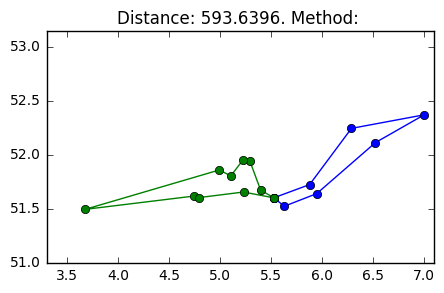In :
save_result(ilp_routes, 1)


### 5. Exercise 2 - NN¶

The nearest neighbor algorithm is used to get an initial solution for the problem. I have implemented the algorithm as follows. It starts at the headquarters and finds the closest city to HQ. If the total distance + distance to closest city + distance from closest city back to HQ is lower than 400km, the city is added to the list of routes. Then that city is updated to the current city and the process repeats itself until adding a new city would result in a route longer than 400km. Each added city is removed from the list of cities that are not visited. After a route is completed the algorithm starts again from HQ.

The following constraints are applied:

• Every route cannot be longer than 400km
• No subroutes are allowed within a route
• All cities in a route are not visited in another route

The result can be seen below.

In :
result_nearest = nearest_neighbor(distances)
plot_routes(result_nearest, 'Nearest Neighbor', figure_size=(10, 5))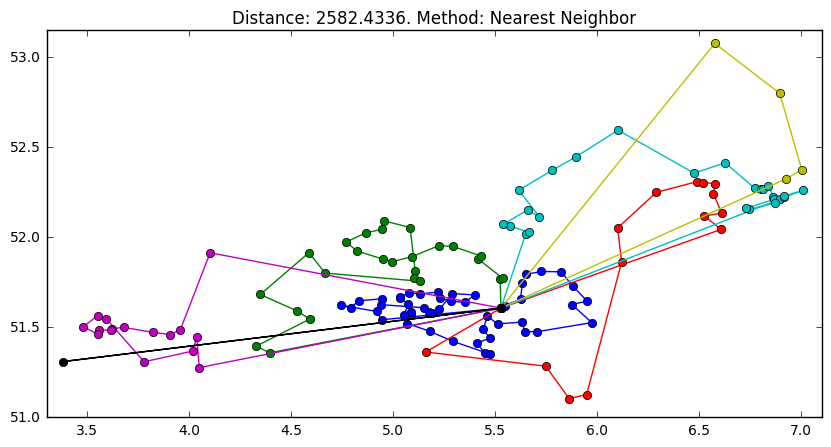### 6 Exercise 3¶

#### 6.1 City Swap¶

City swap is implemented as a within-route consecutive city swap. In other words, it tries to swap consecutive cities in a single route and returns a route as soon as it is better then the starting route. The result is a marginally better result if ran once. However, if ran multiple times you get a significant better result

In :
results_city_swap = {i: city_swap(result_nearest[i]) for i in result_nearest}
plot_routes(results_city_swap, 'City swap', figure_size=(10, 5))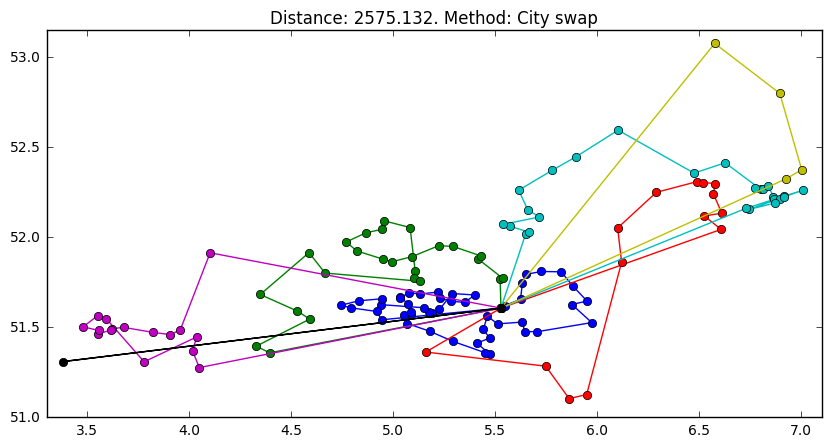### 6.2 City Swap (between routes)¶

In order to further improve the results I implemented a between route version of city swap. This heuristic tries to swap each cities in a route with the closest city in another route. It returns as soon as it finds a solution that is better than the initial solution.

In :
results_city_ext = city_swap_extended(result_nearest)
plot_routes(results_city_ext, 'City Swap Extended', figure_size=(10, 5))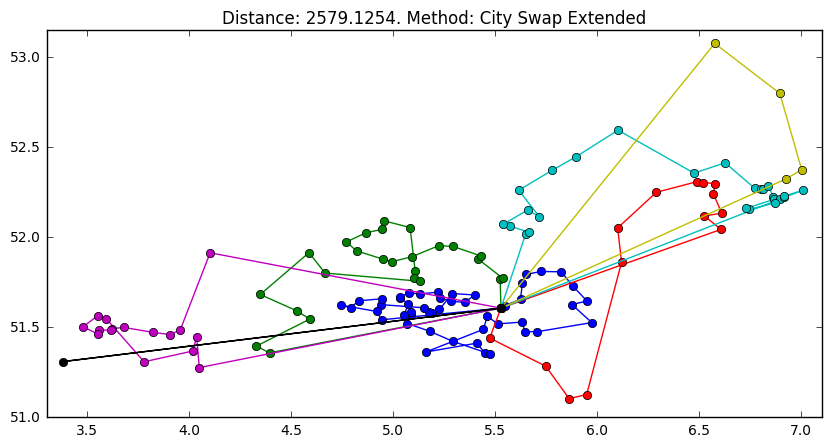### 6.3 Two-opt Exchange (within routes)¶

This version of two-opt exchange work by selecting a subroute within a route and reversing the order. Reversing of the order is done as illustrated below:In :
results_two_opt = {i: two_opt_step(result_nearest[i]) for i in result_nearest}
plot_routes(results_two_opt, 'Two-opt', figure_size=(10, 5))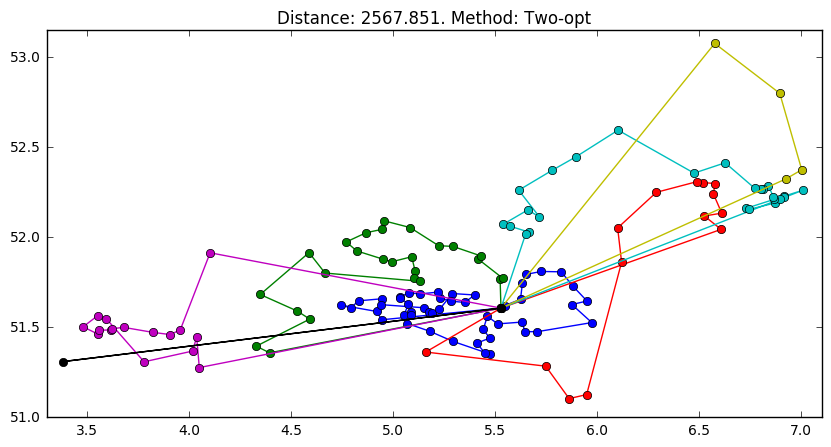### 6.4 Two-opt Exchange (between routes)¶

A single iteration of a two-opt exchange between different routes. The heuristic takes two routes and splits both of them in a random point to create the following routes: 1A, 1B, 2A and 2B. Then, the routes are combined as follows: (1A2A and 1B2B) or (1A2B, 1B2A). The algorithm checks which one is better and returns as soon as the new route is better than the initial route.

In :
results_two_ext = two_opt_step_ext(result_nearest)
plot_routes(results_two_ext, 'Two Opt Between', figure_size=(10, 5))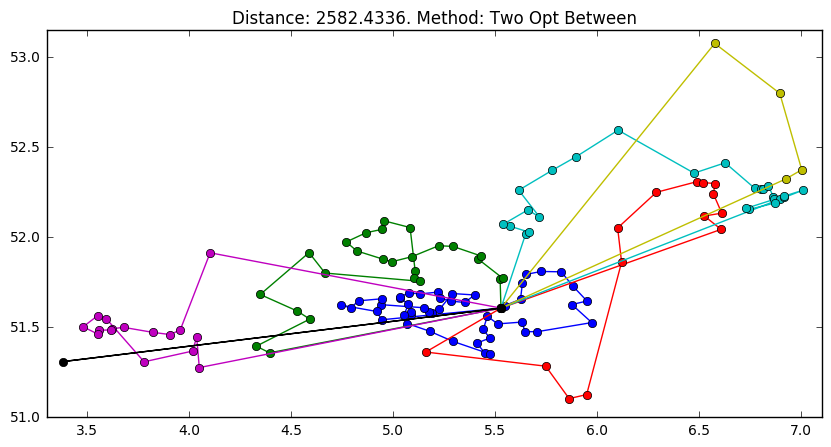### 6.5 Transfer Cities¶

This heuristic tries to move a city from one route to another in order to improve the resulting routes. Implicity, it tries to lower the number of routes as moving a city from a route with a single city reduces the total distance.

In :
results_transfer = transfer_cities(result_nearest)
plot_routes(results_transfer, 'City Transfer', figure_size=(10, 5))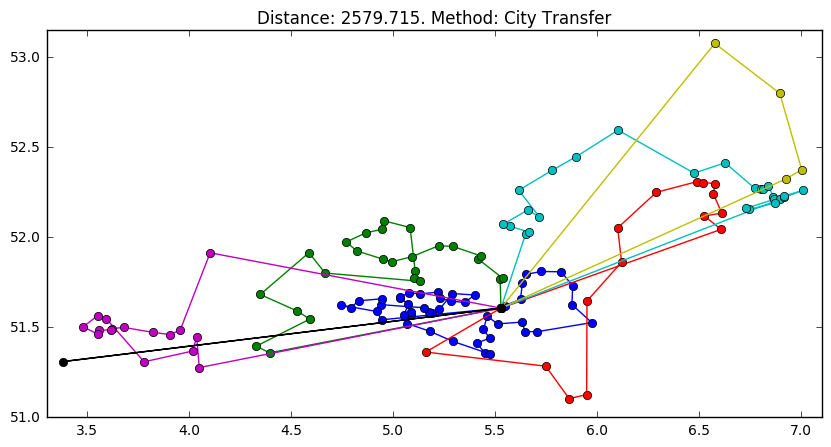### 6.6 Remove Routes¶

This heuristic tries to move all cities in the smallest route to any other routes in order to minimize the number of routes. It allows for a worse solution in order to decrease the number of routes and perhaps explore more possible neighborhoods. It returns as soon as it has moved a city in the smallest route to any other route and if it fits the constraints.

In :
results_remove = remove_routes(result_nearest)
plot_routes(results_remove, 'Remove routes', figure_size=(10, 5))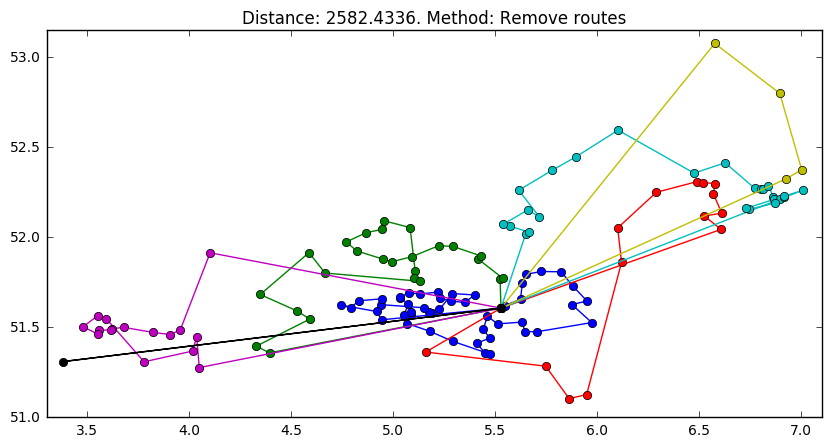### 6.7 Swap Subroutes¶

This heuristic swaps a random subroute of one route with the subroute of another. This is an extension of the two-opt exchange between different routes. It returns as soon as the route is improved

For example:

• route_1 = [0, 1, 2, 3, 4, 5, 6, 0]
• route_2 = [0, 7, 8, 9, 10, 11, 12, 0]

The algorithm may randomly swap subroute [3, 4] in route_1 with subroute [7, 8, 9, 10] in route_2 to get the following results:

• route_1 = [0, 1, 2, 7, 8, 9, 10, 5, 6, 0]
• route_2 = [0, 3, 4, 11, 12, 0]
In :
result_subroutes = swap(result_nearest)
plot_routes(result_subroutes, 'Swap Sub Routes', figure_size=(10, 5))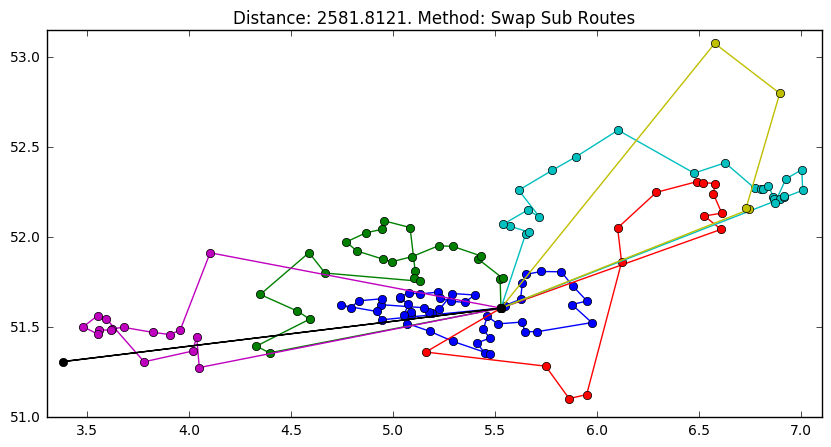An implementation of the tabu search heuristic.

It uses seven heuristics (see functions for descriptions):

* Concecutive city swap (within routes)
* City swap (between routes)
* Two opt exchange (within routes)
* Two opt exchange (between routes)
* Transfer cities
* Remove cities
* Swap Sub Routes



For each iteration tabu search runs the six heuristics and randomly picks a solution. These are random walks that are executed in order to explore neighborhoods that might lead to a better solution:

The randomness of the implementation allows for different neighborhoods to be possible. Moreover, a tabu list is implemented in order to allow the search to go back to an already seen solution and go into a different neighborhood. It returns after a certain amount of iterations if there has been no improvent for 10 consecutive iterations.

Note: Set the number of iterations at 20 if you want quick results. Increasing the number of iterations will obviously result in a better score, but will take a while to run.

In :
tabu, tabu_distances, intermediary_results = tabu_search(result_nearest, 1000, 950)
plot_routes(tabu, 'Tabu', figure_size=(10, 5))

# index_min = np.argmin(tabu_distances) # To get the best result in the list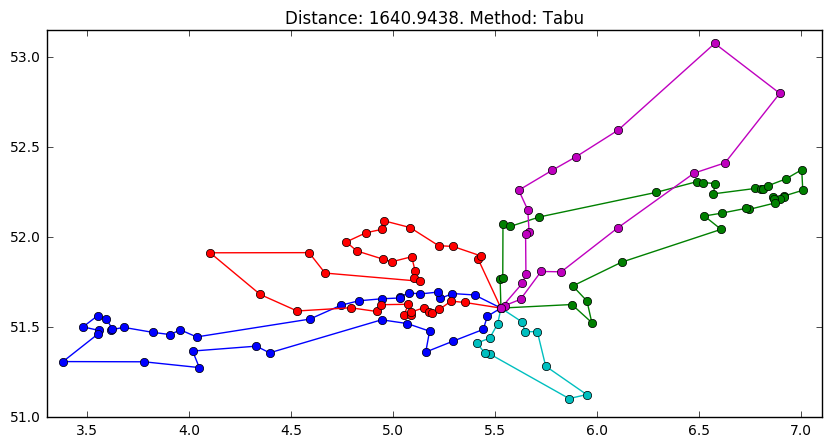In :
fancy_plot(tabu)

Out: